### Quantum Objects

To best illustrate the behavior of quantum objects, we need to investigate the behavior of microscopic particles. We have our choice of particles to choose from: the groundbreaking discoveries that birthed quantum mechanics involved careful study of photons, atoms, and electrons.

Atoms are certainly small enough to behave as quantum objects, but they are often fiendishly complex systems themselves since they are comprised of several different types of subatomic particles, including electrons, neutrons, and protons which all interact in surprising ways. Diving into the deep end with atoms is a recipe for disaster, so let's start simple.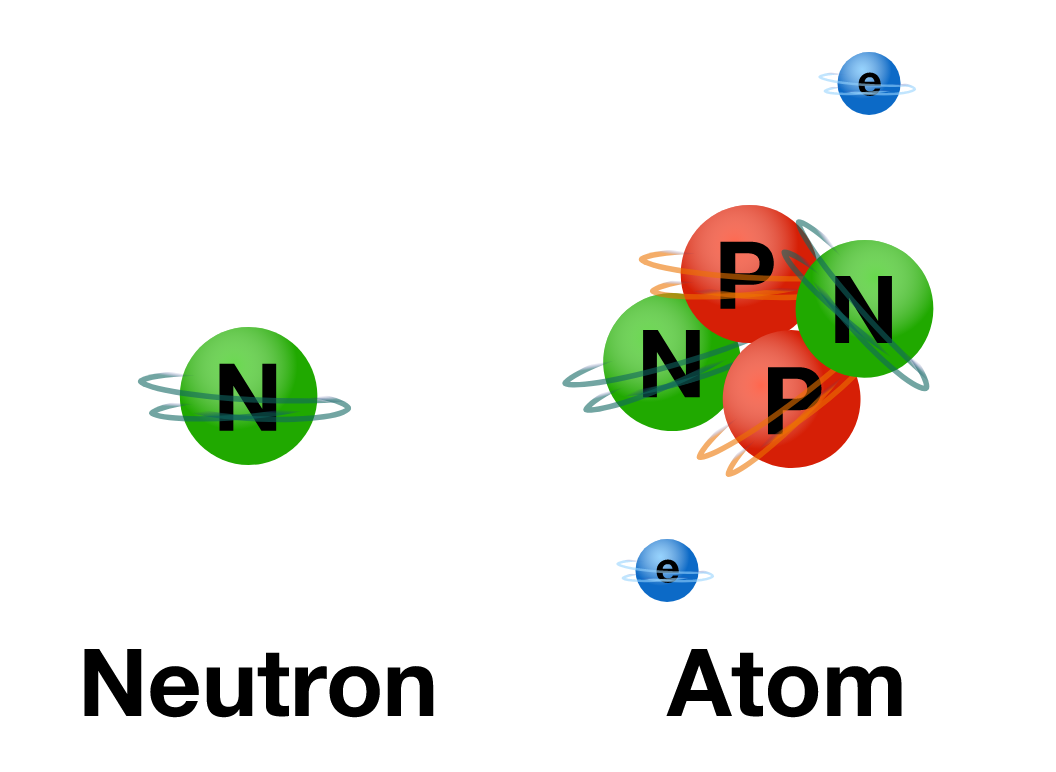In this chapter, we'll study the behavior of neutrons, the most boring of the subatomic particles. Neutrons have no charge, unlike protons and electrons, but have a relatively large mass for a subatomic particle (slightly more than protons).

# Classical Expectations

Understanding how neutrons behave suddenly sprang to the attention of physicists during the Manhattan Project, when it became obvious that neutron transport was critically important for moderating nuclear chain reactions in nuclear power plants and atomic weaponry.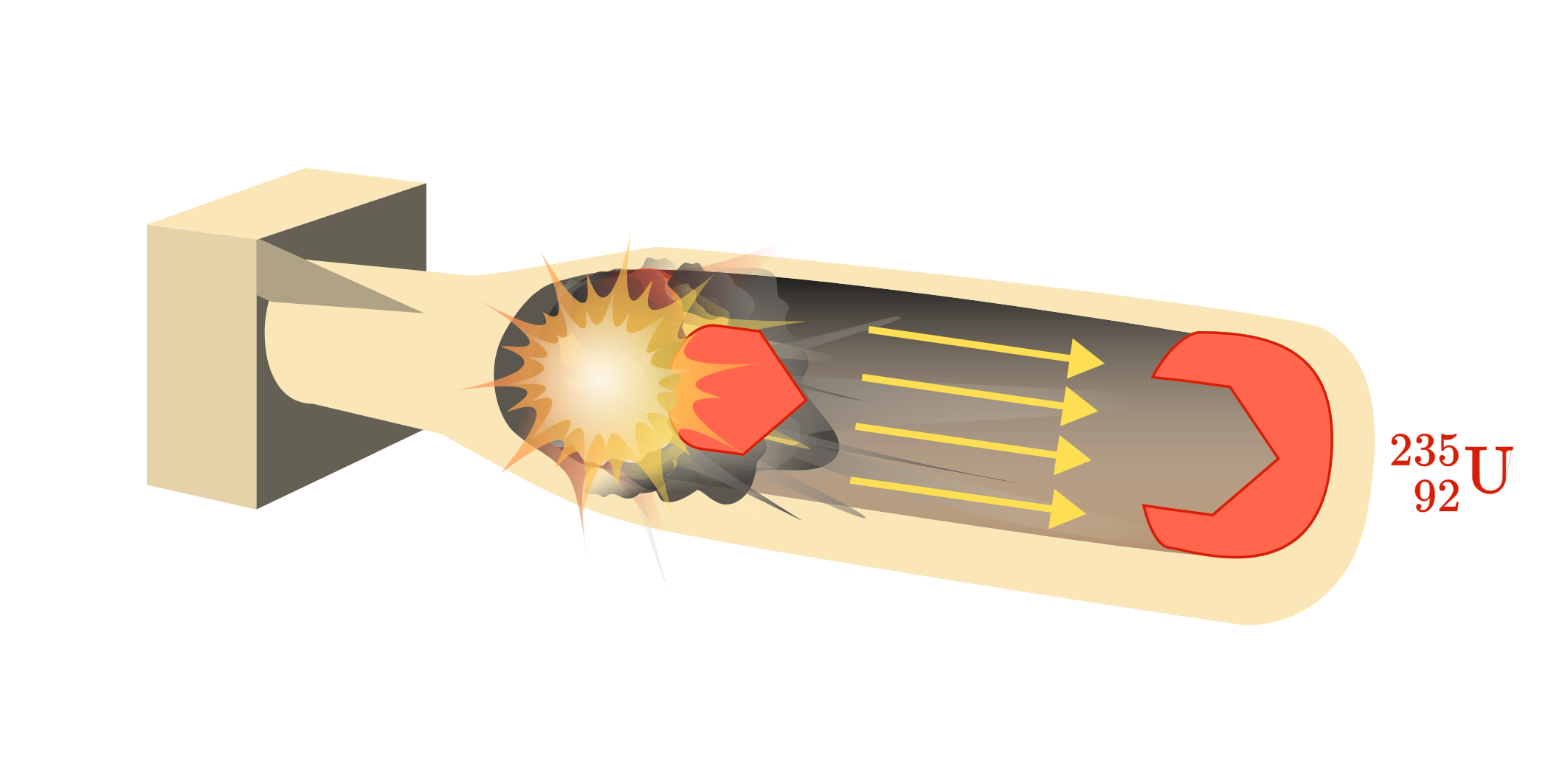Let’s start a bit more simply and consider the behavior of a single neutron:

A single neutron is generated from a sufficiently hot nuclear oven, perhaps as a product of fusion or fission. This neutron exits the oven through an aperture and travels into view. What kind of path do we expect from a ballistic neutron?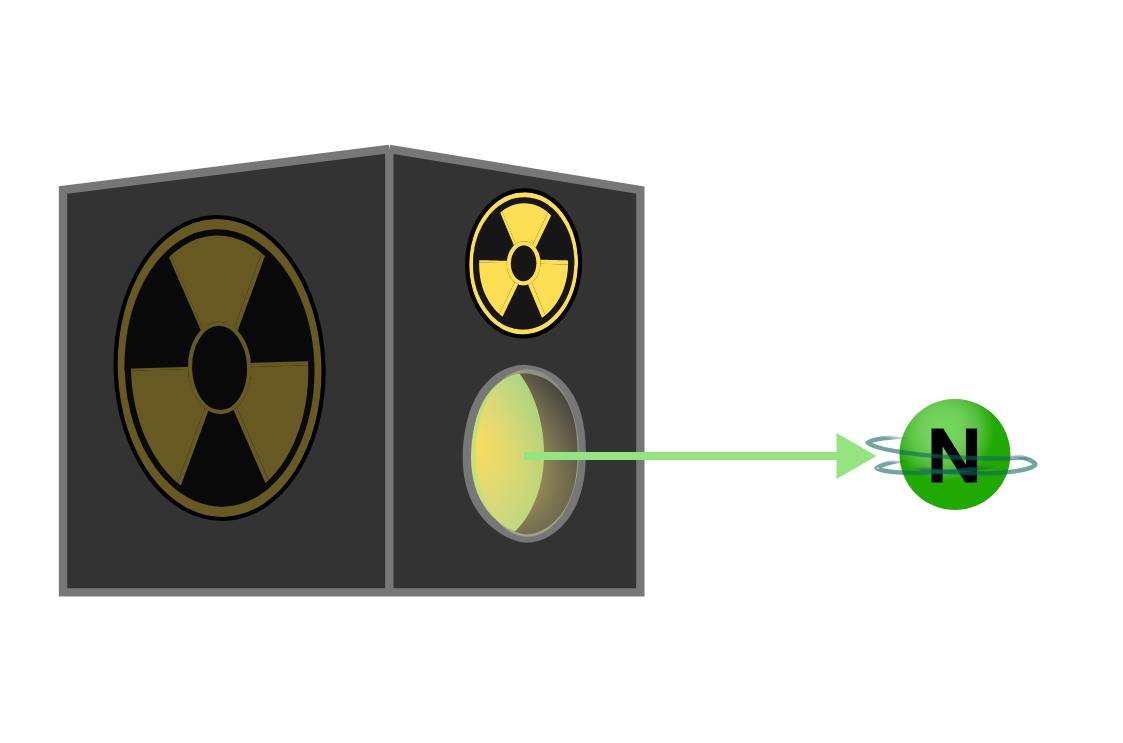# Classical Expectations

The foundations of classical mechanics are still intact: we expect a ballistic neutron to behave much like a fastball or a bullet would in zero gravity as predicted by Newton’s first law. We can neglect the force of gravity on the neutron for our experiments since gravity's acceleration is vanishingly small compared to the velocity of a hot subatomic particle. The neutron will tend to follow a straight trajectory at a constant speed and direction unless acted on by an unbalanced force.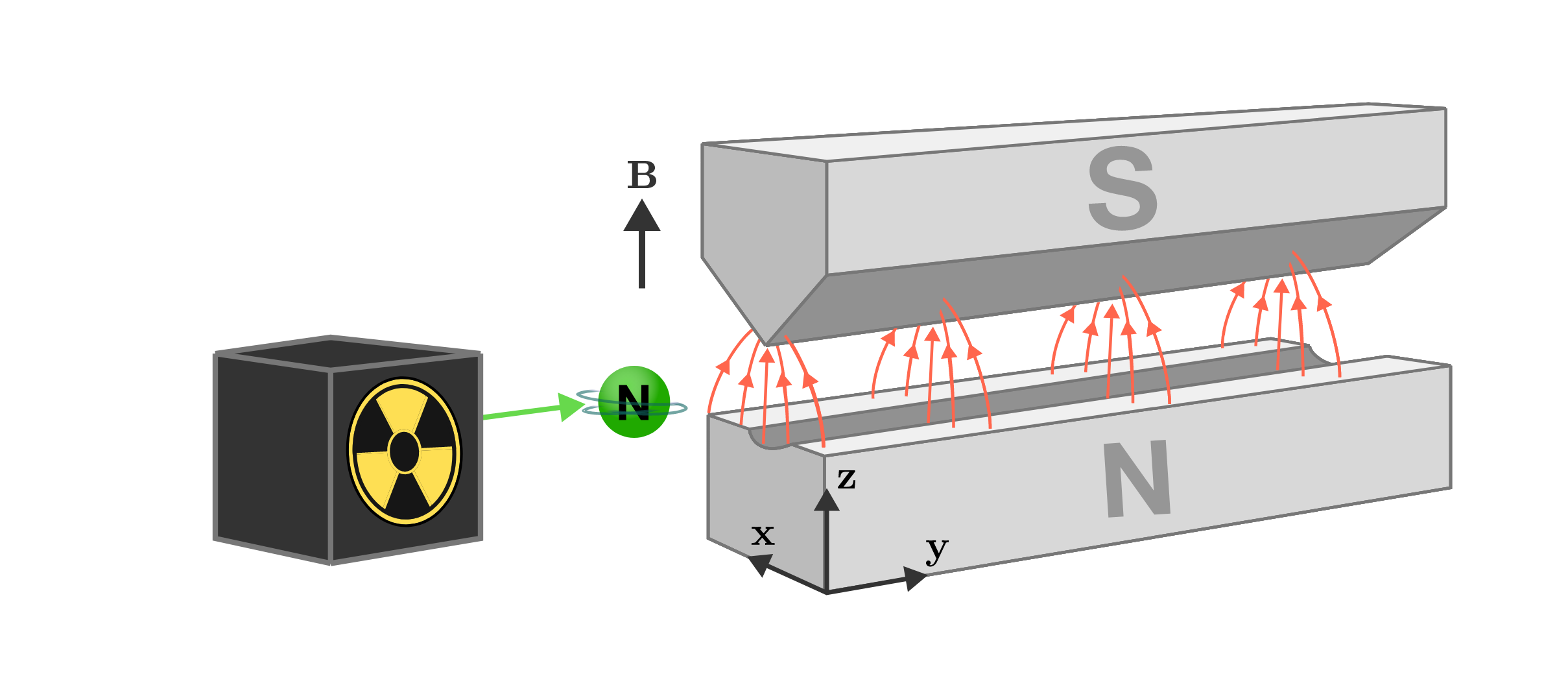Let’s introduce a complication: we arrange two permanent magnets of opposite polarity to generate an inhomogeneous magnetic field $\textbf{B}$ directly perpendicular to the path of our neutron through space.

Given our understanding of classical physics, how might we expect our neutron to be affected by the magnetic field if we treat it as an uncharged point mass?

# Classical Expectations

We’d expect the neutron to behave like any other uncharged mass in a magnetic field: unperturbed.

That isn’t what we observe however: our neutron’s path is deflected much like we might expect from a charged particle (like an electron or a proton), but to a much smaller degree. Detecting this deflection requires a very precise instrument, but it is a reproducible, real effect.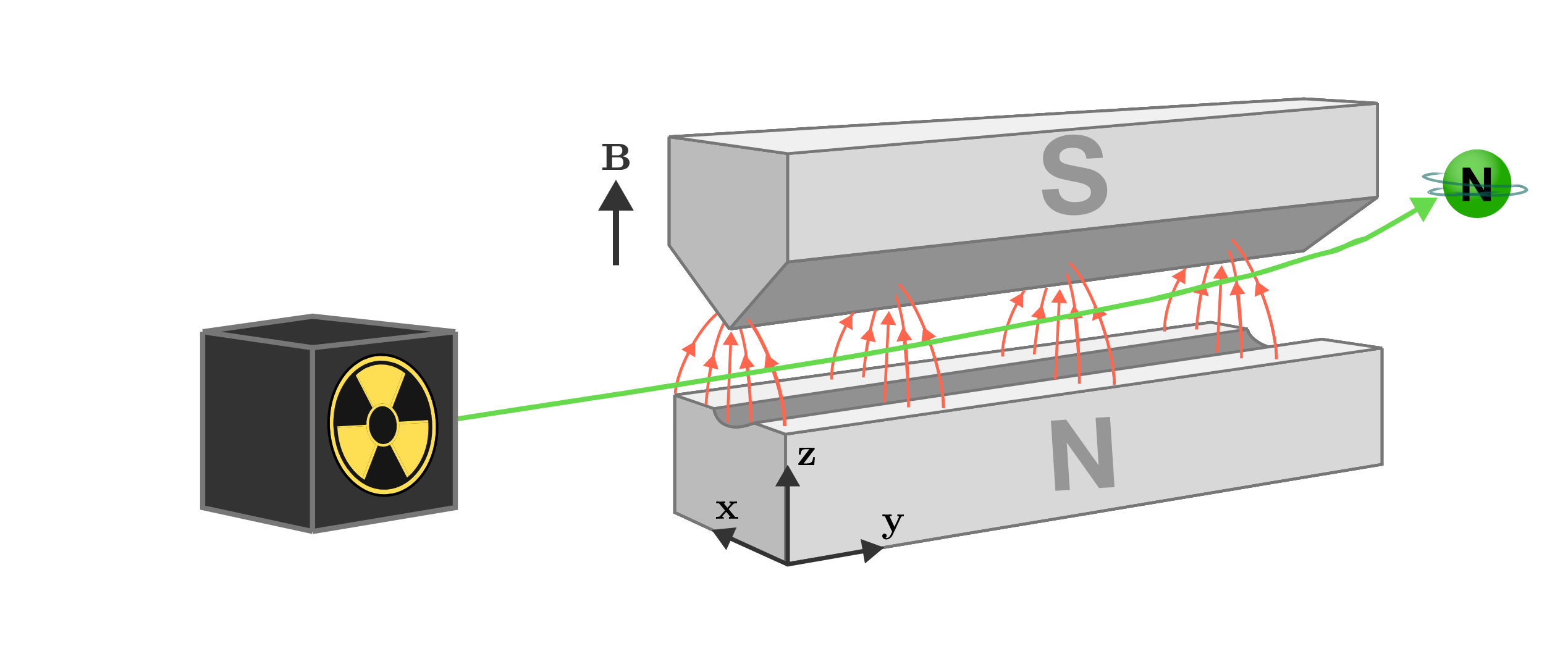Since the neutron is uncharged, this isn’t a deflection caused by a moving charge like you’d observe for an electron. But the deflection implies it has a magnetic moment, just like a charged particle.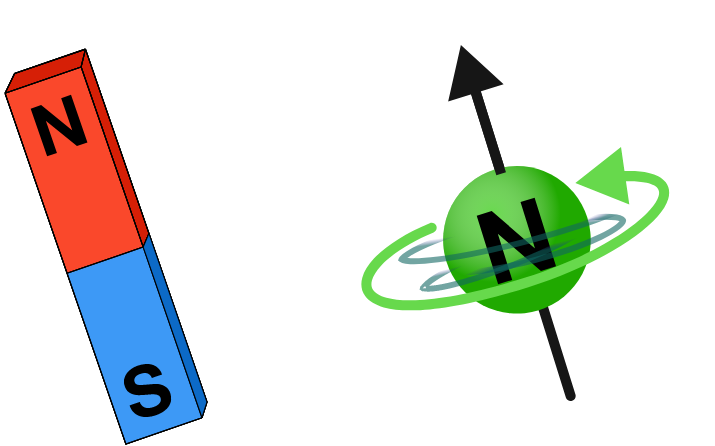Uncharged objects can sometimes have magnetic moments if they are spinning, so experimenters referred to this magnetic moment as a spin magnetic moment $\mu \propto \textbf{S}$. As with any magnetic moment, $\textbf{S}$ has a magnitude and a direction, corresponding to the axis of the spin’s rotation, and behaves like a tiny bar magnet in a magnetic field.

# Classical Expectations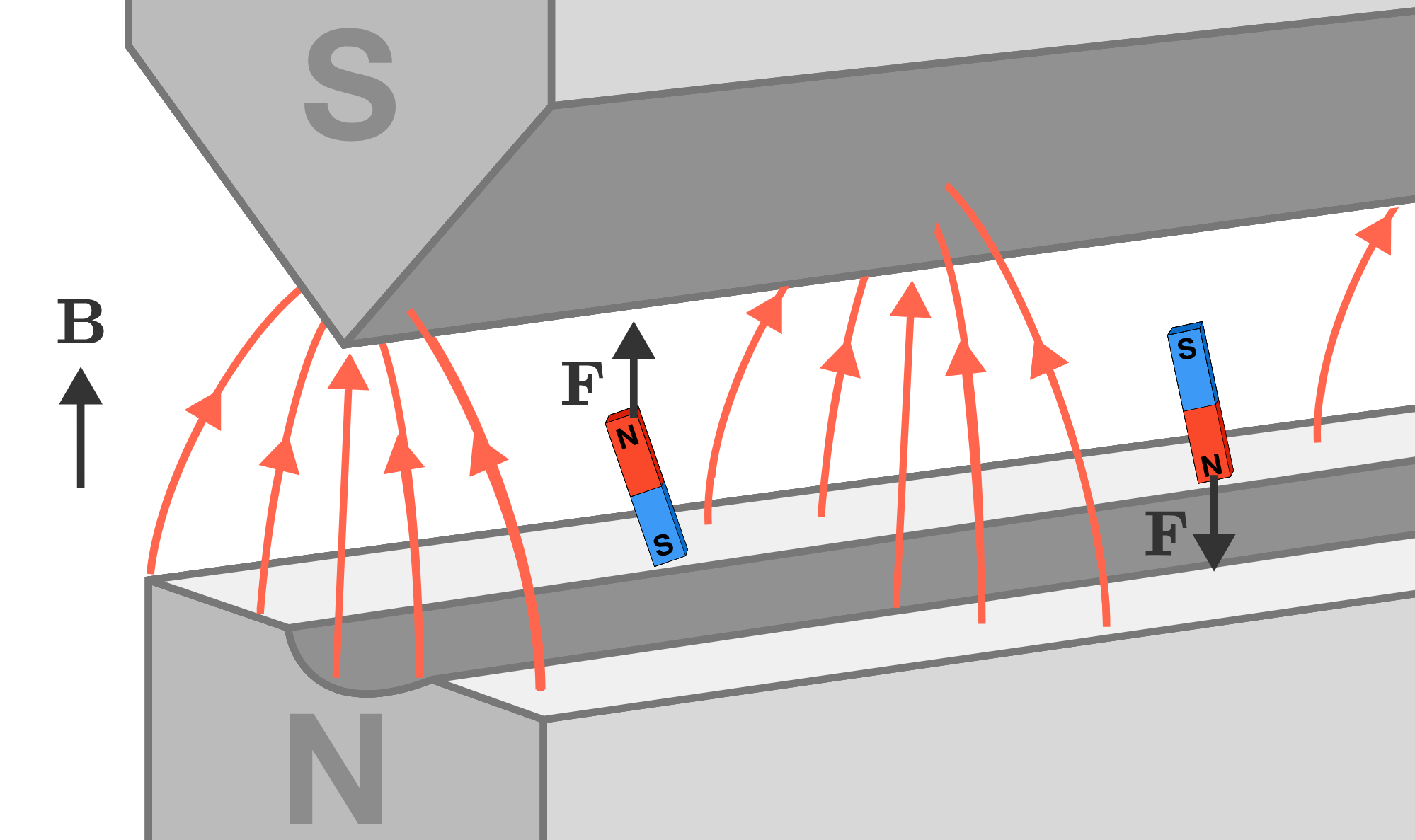A spinning neutron with a magnetic moment $S$ couples with a magnetic field much like a tiny magnet. The magnetic field $\textbf{B}$ depends on the neutron's distance from the apparatus. The interaction between the magnet and the magnetic field also depends on which direction the magnet is pointing; some orientations lead to an upwards deflection, while others cause downwards deflection.

The interaction energy between a spinning neutron or a tiny bar magnet and a magnetic field is given by $U = - \mathbf{S} \cdot \textbf{B}$. Since our apparatus is designed so that the magnetic field $\textbf{B}$ is only along the $z$-axis, the energy is simply given by $U = -\mathbf{S} \cdot \mathbf{B_{z}}.$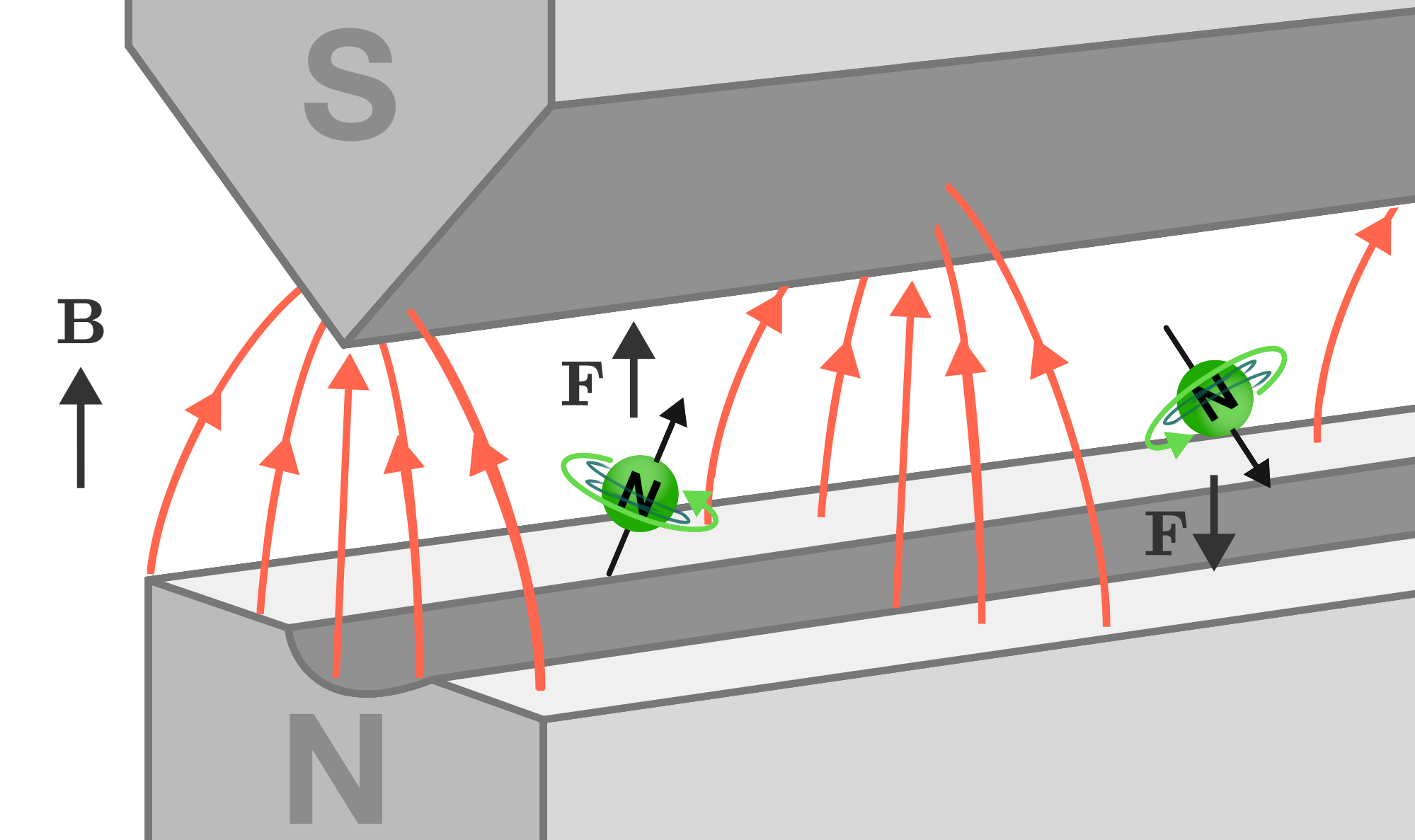How would the deflection force caused by this interaction change if a tiny bar magnet (or a spinning neutron) were flipped $180^{\circ}$ from pointing "up" to pointing "down"?

# Classical Expectations

The force experienced by the neutron depends on the direction of its spin moment since the dot product is equivalent to $F = \frac{\partial }{\partial z} \lvert\mathbf{B}\rvert \lvert \mathbf{S}\rvert \cos{(\theta)},$ where $\theta$ is the angle between the magnetic field and the neutron spin axis.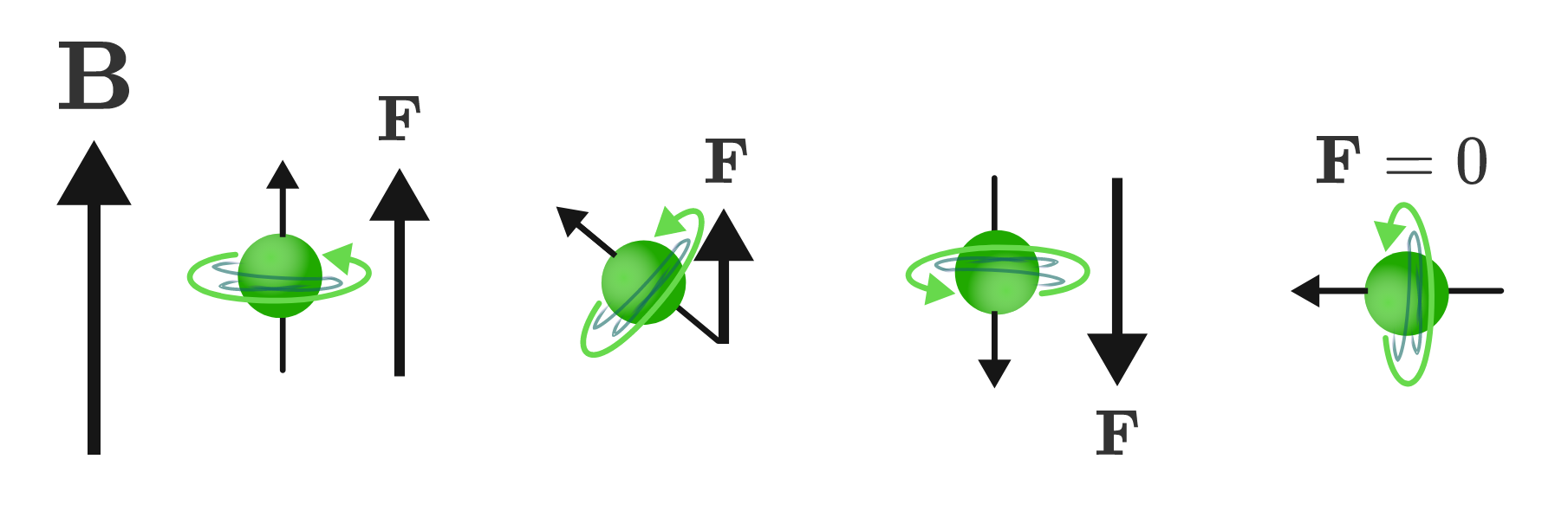If the axis of spin $\mathbf{S}$ is in the same direction as $\mathbf{B}$, then the dot product is positive and the force pushes the neutron into regions of higher magnetic field. However, if $\mathbf{S}$ is in the opposite direction as $\mathbf{B}$, then the dot product is negative, pushing the neutron in the opposite direction. If the neutron just happens to be directly aligned with the $z$-axis of the magnetic field, it would be maximally deflected, while one pointed directly perpendicular (along the $xy$-plane) would not be deflected at all.

If we place a particle detector on the opposite side of the magnet, we can measure the magnitude of the neutron deflection, giving us an indication of which direction its spin is directed.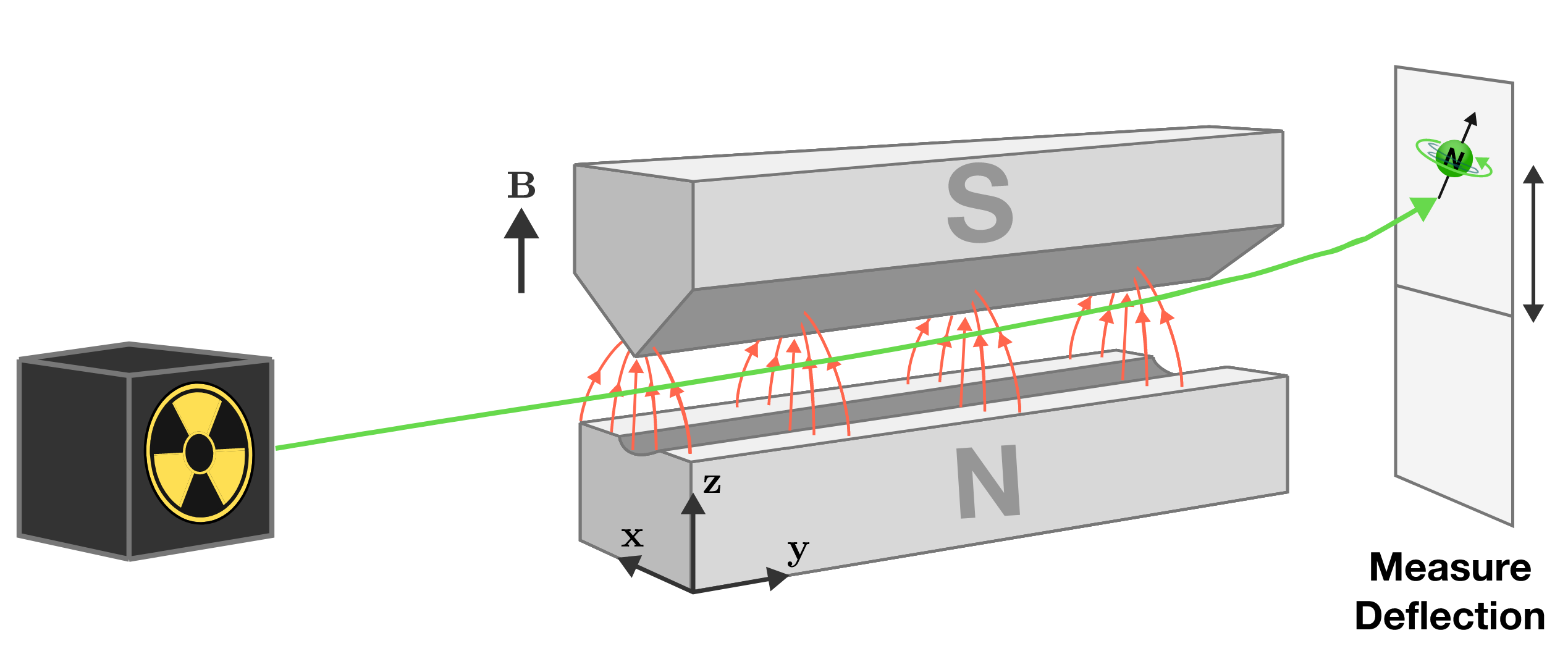# Classical Expectations

Just as soon as we've got the basics down for the behavior of a single neutron, another neutron emerges from the thermonuclear oven, and appears to have the same initial ballistic trajectory as the first.

How do you expect this new neutron to be deflected by the magnet apparatus?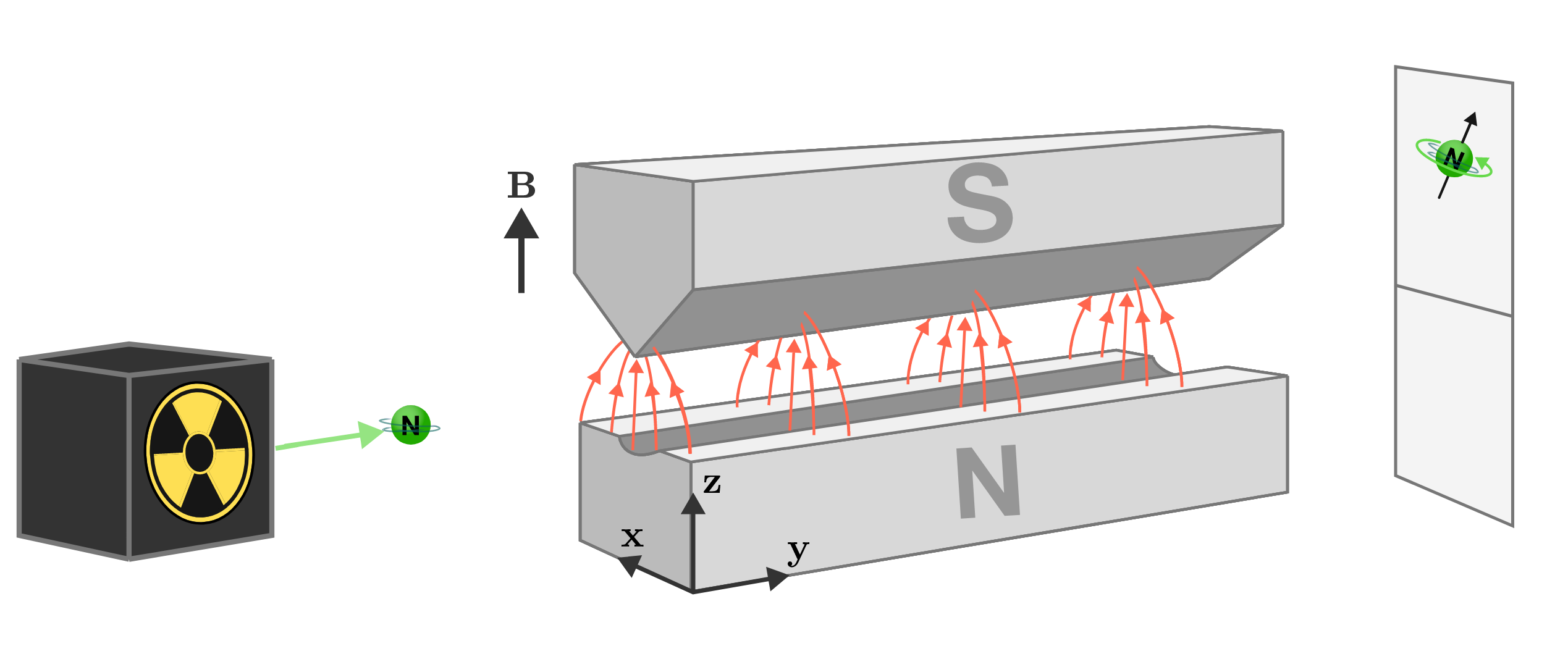# Classical Expectations

Let's turn up the heat in the thermonuclear oven generating our neutrons. Now we’re generating a constant stream of neutrons which emerge from the tiny aperture to our oven pointed in the same direction, headed towards the magnetic field and the detector beyond.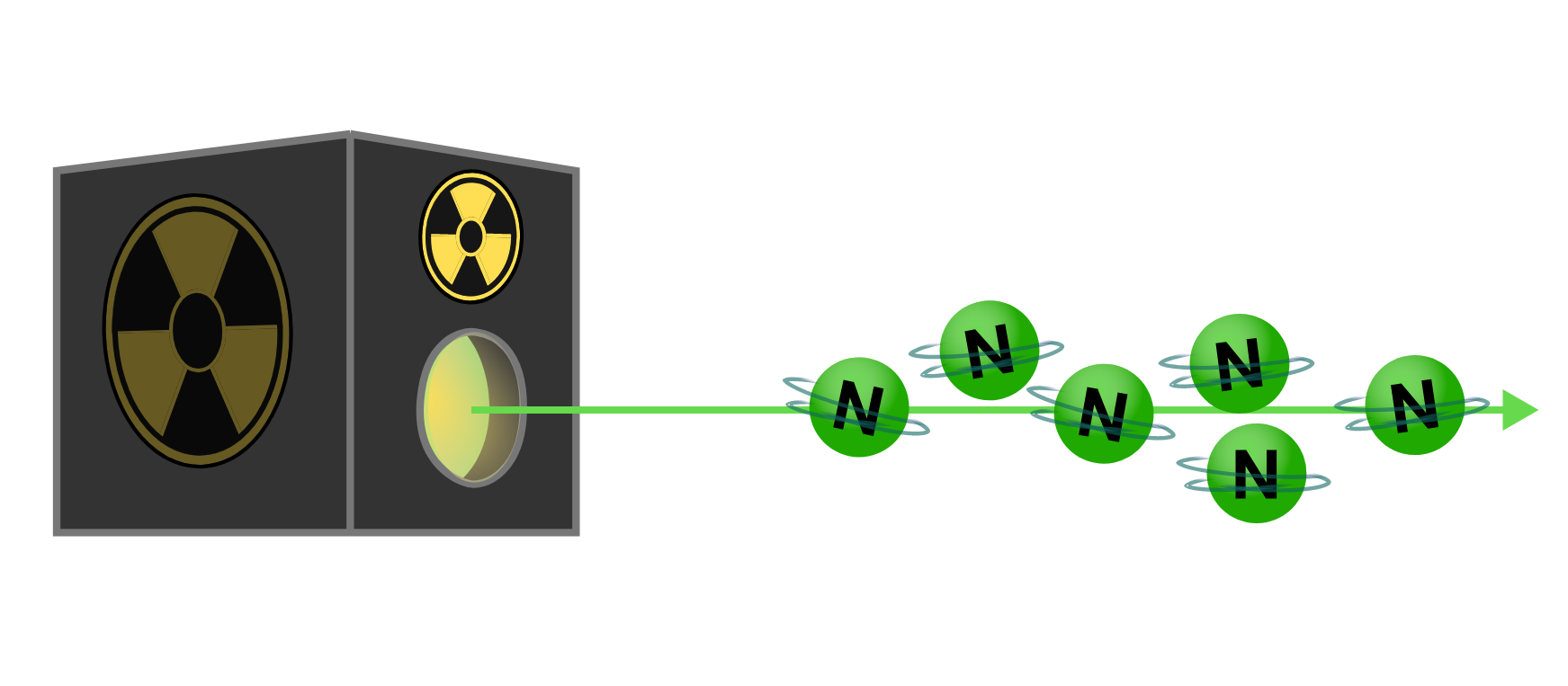Our neutron analyzer measures deflection of neutrons using a magnetic field, with the deflection force depending on the direction of spin. By observing the neutron deflection, this analyzer is really measuring $\mathbf{S_z}$, the part of the spin axis which aligns with the applied magnetic field in the $z$-direction.

What would we expect the distribution of a constant stream of neutrons to look like on our detector screen?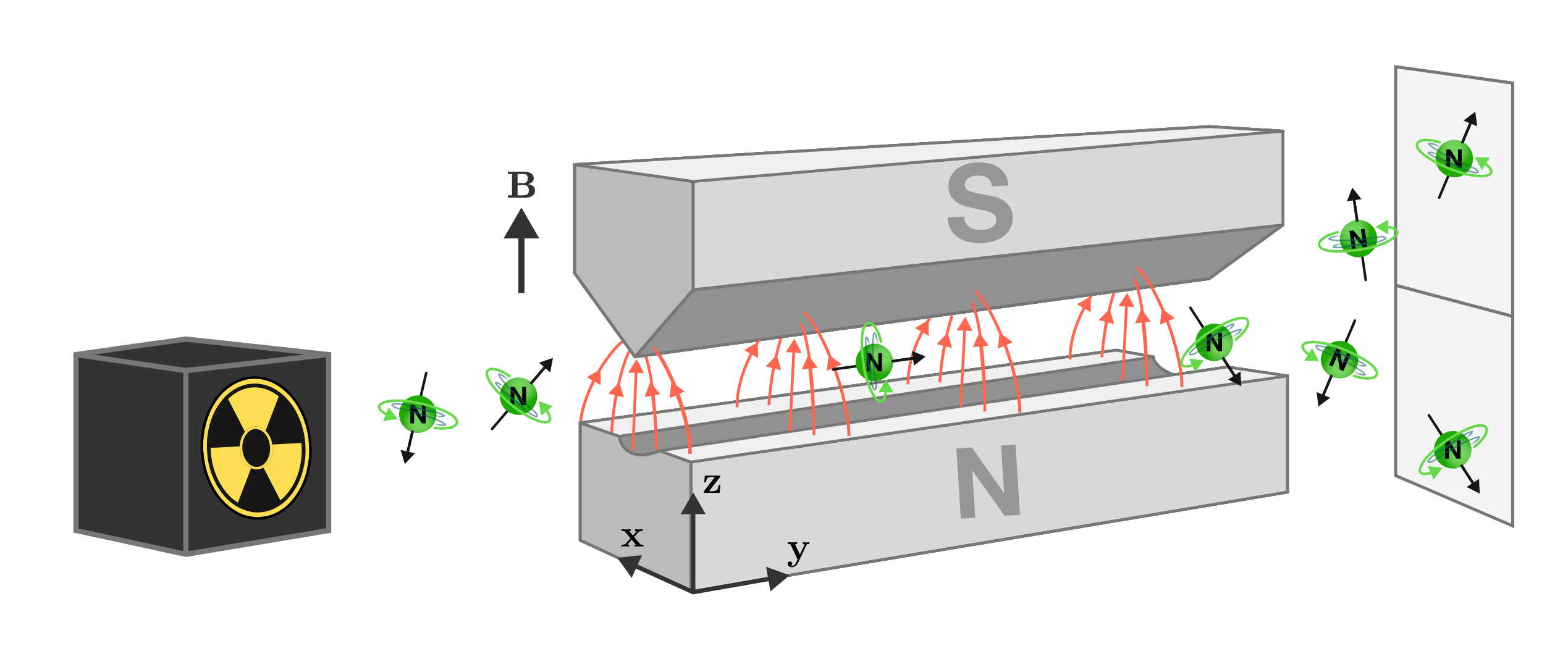# Classical Expectations

A classical neutron can point in any direction at all, with no preferred orientation. Randomly oriented neutrons would be broadly spread across the detector, centered at no deflection.

Neutron spin is not behaving like a classical property: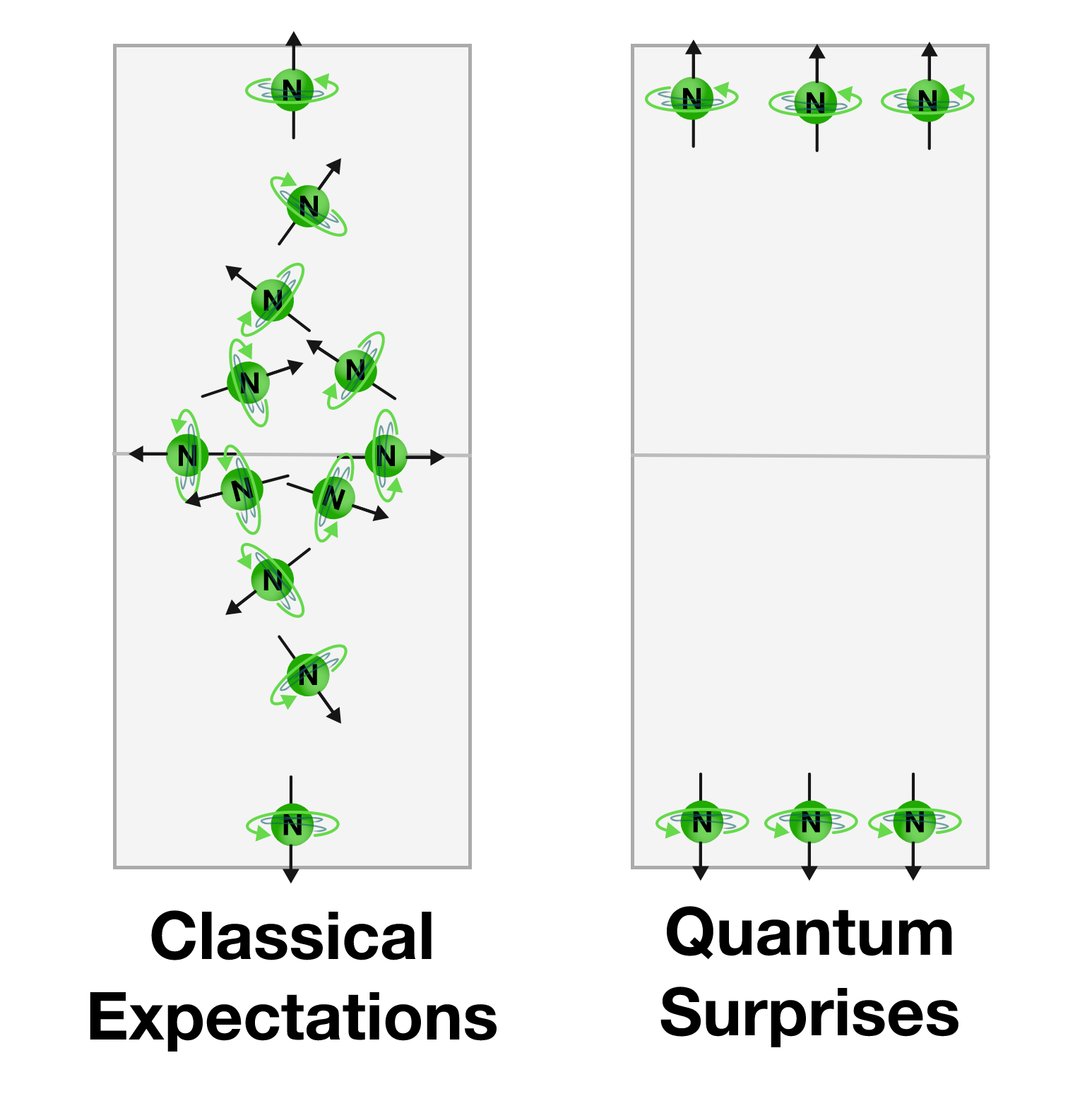We don't see a broad spread of randomly oriented neutrons. We see two beams: one oriented up on the $z$-axis, and one oriented down. According to our analyzer, neutrons are only found in two possible states out of the infinite number of random orientations they could have.

Spin must be a quantum property because it is quantized, meaning it is only allowed to have certain values. Please leave your classical expectations at the door.

# Classical Expectations

Why is this happening? What's so special about neutrons that they have to be quantum while bar magnets and baseballs happily follow classical expectations?

Well, we chose neutrons for our case study because classically they shouldn't be affected at all by a magnetic field. But they are deflected in this strange binary way because they have a quantum property that we call spin. It's important to note that the neutron isn't spinning—at least not in any classically meaningful sense. Spin is a property that every particle has, but its effects are very small.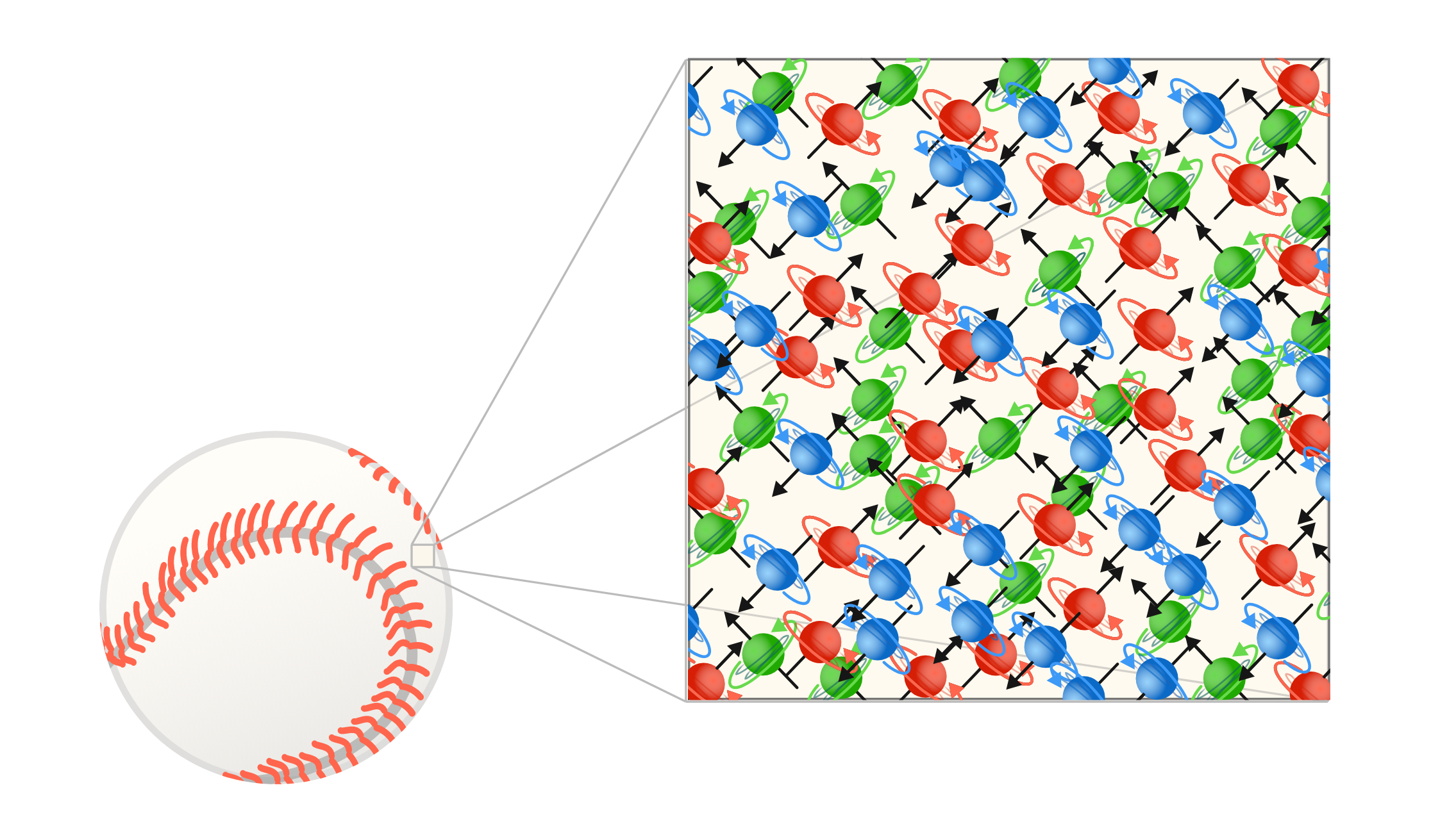At the human scale, objects are composed of extraordinarily large numbers of microscopic quantum objects; including neutrons, protons, and electrons. Why don't we observe the binary quantum effects of spin in macroscopic objects?

# Classical Expectations

At the human scale, binary quantum effects like spin are averaged over incredibly large numbers of microscopic objects, and tend to smooth out to reproduce continuous classical behavior.Only extremely precise measurement of isolated quantum objects can observe the effects of spin. The strength of the neutron spin magnetic moment is about $10^{34}$ times lower than the strength of bar magnets you're used to. This is why quantum mechanics was discovered by a bunch of tenacious physicists who were obsessed with the details past the sixth place of decimals.

# Classical Expectations

×